Courses
Courses for Kids
Free study material
Free LIVE classes
More

# NCERT Solutions for Class 6 Maths Chapter 4 - Basic Geometrical IdeasLIVE
Join Vedantu’s FREE Mastercalss

## NCERT Solutions for Class 6 Maths Chapter 4 - Basic Geometrical Ideas

Class 6 Maths Chapter 4 Basic Geometrical Ideas is a prime part of the syllabus that constructs the fundamental concepts related to various geometrical elements and figures among students. To understand the context and concepts of this chapter, download and refer to the NCERT solutions designed by the subject experts of Vedantu. Find out how the fundamental questions in the exercises of this chapter have been answered and learn the skills to score more in the exams.

NCERT Solutions of Class 6 Maths Chapter 4 Basic Geometrical Ideas offered by Vedantu assist students in understanding Geometry. These solutions of Vedantu provide a strong foundation from the beginning of each concept of Mathematics. The number of examples available in the PDF books makes the students very strong and confident on all topics. Every NCERT Solution is provided to make the study simple and interesting on Vedantu. Subjects like Science, Maths, English, and Hindi will become easy to study if you have access to NCERT Solution for Class 6 Science, Maths solutions and solutions of other subjects.

NCERT Maths book Class 6 Chapter 4 solutions are available on the official website of Vedantu and are very easy to understand for the students. After reading the concept given in the textbook, practise solved examples. If the students have any doubts, the students can post their doubts in the chat box. It is the ultimate advantage of NCERT solutions. Downloading PDFs is free of cost so that the students can practise some in their convenient and leisure times.

Last updated date: 27th Sep 2023
Total views: 455.7k
Views today: 4.55k

## Access NCERT Solutions for Class 6 Maths Chapter 4 - Basic Geometrical Ideas

Exercise: 4.1

1. Use the given figure to name the following: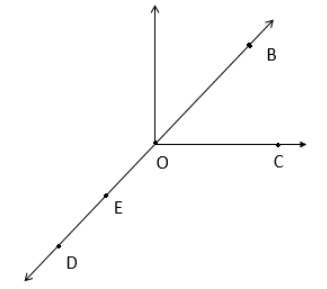a. Five points

Ans: A point denotes a location.

O, B, C, D, E are the five points.

b. A line

Ans: When the line segment from D to B is extended beyond D in one direction and beyond B in another direction, without any end, we get a line $\overleftrightarrow{DB}$

A line $\overleftrightarrow{DB}$

c. Four rays

Ans:  A portion of a line that starts at one point and goes endlessly in a direction is called a ray.

Rays are $OD,\:OE,\:OC,\:OB$

d. Five-line segments

Ans: The shortest join of two points represents a line segment.

Line segments are $\overline {DE}, \overline {OE,} \overline {OC} ,\overline {OB} ,\overline {OD}$

2. Observe the given line. Choose only two letters at a time from the four given and name it in all possible twelve ways.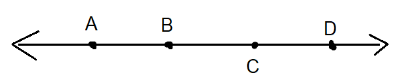Ans: The shortest join of two points represents a line segment.

$\overline {AB} ,\overline {AC} ,\overline {AD} ,\overline {BC} ,\overline {BD} ,\overline {CD} ,\overline {BA} ,\overline {CA} ,\overline {DA} ,\overline {CB} ,\overline {DB} ,\overline {DC}$

3. Use the figure to name:

(Image will be updated soon)

a. A line containing a point $E$

Ans: A line containing $E$ is $\overline {AE}$or $\overline {FE}$

b. Line passing through $A$

Ans: A line passing through $A$ is $\overline {AE}$

c. The line on which $O$ lies

Ans: A line on which $O$ lies is $\overline {OC}$

d. Two pairs of intersecting lines

Ans: If two lines have one common point, they are called intersecting lines.

Two pairs of intersecting lines are $\overline {AD} ,\overline {CO}$ and $\overline {AE} ,\overline {FE}$

4. How many lines can pass through:

a. One point

Ans: An infinite number of lines. An infinite number of lines pass through point A.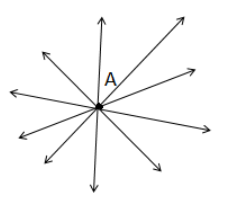b. Two given points

Ans: Consider the two points D and B. Only one line can pass through these two points.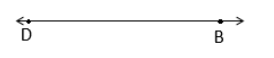Hence, through two given points only one line can pass.

5. Draw a rough picture and label each of the subsequent cases:

a. Point P lies on $\overline {AB}$

Ans:

(Image will be updated soon)

b. $\overline {XY}$ and $\overline {PQ}$ intersect at M.

Ans:

(Image will be updated soon)

c. A line named ‘l’ contains E and F but not D

Ans:

(Image will be updated soon)

d. OP and OQ meet at O

Ans:

(Image will be updated soon)

6. Consider the figure below of the line $\overline {MN}$. Identify if the given statements are true or false based on the given figure:

(Image will be updated soon)

a. On the line, $\overline {MN}$, points are M, Q, O, N, P

Ans: True

A line consists of many points and a point determines a location. The line $\overline {MN}$ is obtained when points M, N are extended indefinitely on both sides. M, Q, O, N, P are hence the points on it.

b. The points on a line segment $\overline {MN}$ are M, O, N

Ans: True

The line segment represents the shortest join of two points. Here M, N are the endpoints of the line segment $\overline {MN}$ and we consider the point only till the endpoint of this segment $\overline {MN}$. So, M, O, N are the points.

c. M and N are endpoints of the line segment $\overline {MN}$

Ans: True

The line segment represents the shortest join of two points. Here M, N are the endpoints of the line segment $\overline {MN}$

d. O and N are endpoints of the line segment $\overline {OP}$

Ans: False

The line segment represents the shortest join of two points. For the line segment $\overline{OP}$, O and P, are endpoints.

e. M is one of the endpoints of the line segment $\overline {QO}$

Ans: False

For the line segment $\overline {QO}$, Q and O are the endpoints. The shortest join of two points is represented by a line segment.

f. Point M is a point on ray OP

Ans: False

A ray starts at a starting point and goes endlessly in a direction. For the ray OP, O is the starting point and the direction of the ray is towards P. M is hence not a point on ray OP.

g. Ray QP is different from ray OP

Ans: True

For the ray QP, Q is the starting point and for the ray OP, O is the starting point. So, both these rays are different.

h. Ray OP same as ray OM

Ans: False

A ray starts at a starting point and goes endlessly in a direction. For the ray OP and OM, though the starting points are the same, the direction of both rays is different.

i. The ray OM is not opposite to the ray OP.

Ans: False

For the ray OP and OM, the starting point is O. But the direction of ray OM is towards M, and the direction of OP is towards P. These points are on the same line and the ray OM is opposite to ray OP.

j. The initial point of $\overline {OP}$ is not O.

Ans: False

For the line segment $\overline{OP}$, O and P are the two endpoints and O is the initial point.

k. Point N is the initial point of  $\overline {NP}$ and $\overline {NM}$

Ans: True

The line segment represents the shortest join of two points. For both of the line segments $\overline{NP}$ and $\overline{NM}$, N is the initial point.

Exercise: 4.2

1. Categorize the following curves as ‘Open’ or ‘Closed’

a.

(Image will be updated soon)

Ans: Open

b.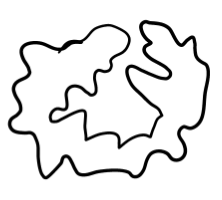Ans: Closed

c.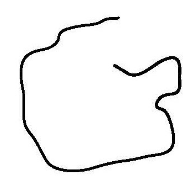Ans: Open

d.

(Image will be updated soon)

Ans: Closed

e.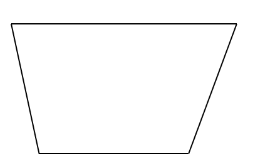Ans: Closed

2. Draw rough figures to illustrate the following cases:

a. Open curve

Ans: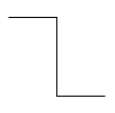b. Closed curve

Ans: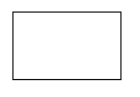3. Draw any polygon and also shade its interior.

Ans: Polygon ABCDE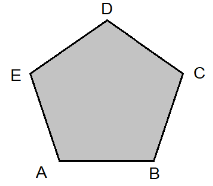4. Analyze the figure and answer the questions: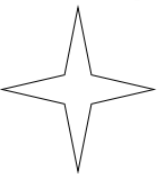a. Is it a curve?

Ans: Ye

b. Is it closed?

Ans: Yes

5. Illustrate each one mentioned below with a rough diagram:

a. Draw a closed curve that is not a polygon.

Ans: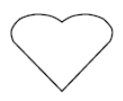b. Draw an open curve that is made up entirely of line segments.

Ans: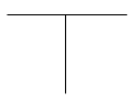c. A polygon with two sides.

Ans: A polygon having two sides is impossible.

Exercise: 4.3

1. Identify and name the angles in the given figure: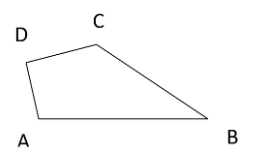Ans: Starting from a common starting point, an angle is made up of two rays. So, here

$\angle ABC,\angle CDA,\angle DAB,\angle DCB$ are the names of angles.

2. Consider the given diagram and name the point(s) for the given cases:

(Image will be updated soon)

a. In the interior of $\angle DOE$

Ans: A given angle can lead to three divisions of a region. The region on the angle, the region interior of angle, and region exterior of angle. The points interior of the angle $\angle DOE$ is the interior points.

Point interior of $\angle DOE$ is A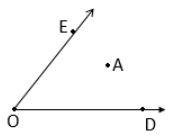b. In the exterior of $\angle EOF$

Ans:   Region exterior of the angle $\angle EOF$ is the exterior region and points in this region are exterior points.

Points exterior of $\angle EOF$ are C, A, D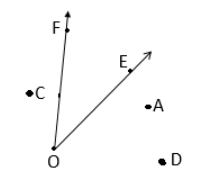c. On $\angle EOF$

Ans: A given angle can lead to three divisions of a region and some of the points may be located on the angle.Points on $\angle EOF$ are E, B, O, F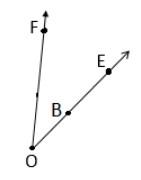3. Plot a rough diagram of two angles such that they have:

a. One point in common

Ans:

(Image will be updated soon)

$\angle AOB$ and $\angle DOC$ have O as their common point.

b. Two points in common

Ans:

(Image will be updated soon)

$\angle ABC$ and $\angle CBE$ has B and C as their two common points.

c. Three points in common

Ans:

(Image will be updated soon)

$\angle ABC$ and $\angle CBE$ have B, D, C as their three common points.

d. Four points in common

Ans:

(Image will be updated soon)

$\angle ABC$ and $\angle CBE$ have B, F, D, C as their four common points.

e. One ray in common

Ans:

(Image will be updated soon)

$\angle AOB$ and $\angle AOC$ has the ray $\overline {AO}$in common.

Exercise: 4.4

1. Sketch a triangle ABC. Mark a point Q in its exterior and a point P in its interior. Is point A located in the exterior or the interior of the triangle?

Ans:

(Image will be updated soon)

A triangle has got an exterior and an interior region. Point Q is in its exterior and points P in its interior. The point ‘A’ is neither located interior nor exterior of the triangle. ‘A’ is the vertex and lies on the triangle ABC.

2. Analyze the given figure and answer the questions.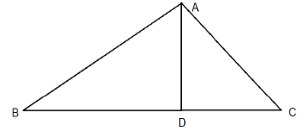a. Identify three triangles in the figure

Ans:  A three-sided polygon is a triangle.

The three triangles are $\Delta ABC,\Delta ABD,\Delta ADC$.

b. Write the names of seven angles.

Ans: Angles are $\angle ADB,\angle ADC,\angle ABD,\angle ACD,\angle BAD,\angle CAD,\angle BAC$

(Image will be updated soon)

(Image will be updated soon)

(Image will be updated soon)

(Image will be updated soon)

(Image will be updated soon)

(Image will be updated soon)

c. Name the six-line segments.

Ans: The line segment represents the shortest join of two points.

Line segments are $\overline {AB} ,\overline {AC} ,\overline {AD} ,\overline {BD} ,\overline {DC} ,\overline {BC}$

d. Which two triangles have $\angle B$ as common?

Ans: A common angle is an angle common to both the given triangle.

Consider the $\Delta ABC,\Delta ABD$. Here $\angle B$ is common to both the triangles.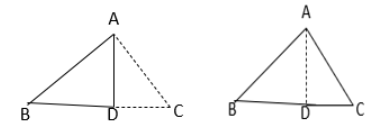Triangles having common $\angle B$ are $\Delta ABC,\Delta ABD$

Exercise: 4.5

1. Sketch of a quadrilateral PQRS. Draw its diagonals. Name them. Determine for the given quadrilateral, if the meeting point of the diagonals is in the exterior or interior?

Ans:

(Image will be updated soon)

The diagonals PR and SQ meet inside the quadrilateral at O. This point “O” is located inside the quadrilateral, So the meeting point is in the interior of the quadrilateral.

2. Draw a quadrilateral KLMN. State:

a. Two pairs of opposite sides

Ans: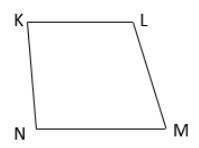A quadrilateral is a four-sided polygon. Opposite sides are sides opposite to each other. One pair of opposite sides is KL and NM and another is KN and LM

b. Two pairs of opposite angles

Ans: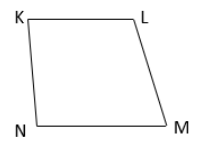The two angles of a quadrilateral that are not adjacent to each other are called opposite angles. Pair of opposite angles are $\angle K$ and $\angle M$, and  $\angle L$  $\angle N$

c. Two pairs of adjacent sides

Ans: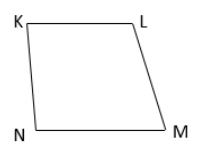If two sides of a quadrilateral have a common endpoint, then they are the adjacent sides. The pair of adjacent sides are KN and NM, KL and LM.

d. Two pairs of adjacent angles

Ans: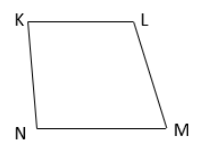If two angles of a quadrilateral have a common side, then they are called adjacent angles. The pair of adjacent angles are $\angle K$ and $\angle N$, $\angle L$ and $\angle M$

3. Investigate:

Make a triangle and a quadrilateral with the use of strips and fasteners.

From any one of the vertices of the triangle, slightly push inward. Do the same to the quadrilateral. Is the triangle distorted? Is the quadrilateral distorted? Is the triangle rigid?

Why do electric towers make use of triangular shapes and not quadrilateral shapes?

Ans: On moving the vertex of the triangle, the whole triangle moves, but the shape remains the same. So, the triangle is rigid.

On moving the vertex of the quadrilateral, the shape of the quadrilateral becomes distorted.

Since on moving the ends of the triangle, the shape remains the same, that is why structures like electric towers use triangle shape.

Exercise: 4.6

1. From the figure, identify:

(Image will be updated soon)

a. The centre of the circle

Ans: The centre of a circle is a fixed point. The path followed from this fixed point at the same distance forms a circle.

Here the centre of the circle is O

Ans: The line segment joining the centre of the circle to a point on the given circle forms the radius.

Radii are OA, OB and OC

c. A Diameter

Ans: A diameter of a circle is a chord passing through the centre of the circle.

The diameter here is AC

d. A chord

Ans: A chord of a circle connects two points on a circle.

A chord here is ED

e. Two points in the interior

Ans: Interior points are points located in the inside region of the circle and here interior points are O, P

f. A point in the exterior

Ans: The points located in the exterior region of the circle are the exterior points. Here the exterior point is Q

g. A sector

Ans: A sector is bounded by an arc on one side and a pair of radii on the other two sides.

A sector is OAB

h. A segment

Ans: The interior region of the circle, bound by an arc and a chord is a segment of a circle.

A segment is ED

a. For a circle, is each diameter also a chord?

Ans:  A chord of a circle connects two points on a circle.

A diameter of a circle is a chord passing through the centre of the circle.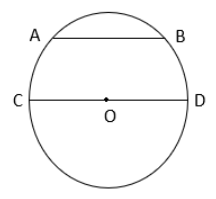Here, AB and CD are chords. CD is the chord passing through the centre of the circle and it is called the diameter.

So, for a circle, every diameter is also a chord.

b. For a circle, are all chords also a diameter?

Ans:  A chord of a circle connects two points on a circle.

Diameter is the largest chord passing through the centre of the circle.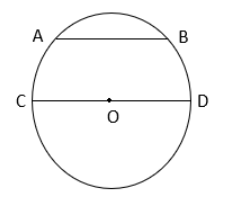Here, AB and CD are chords. CD is the largest chord passing through the centre of the circle and it is called the diameter.

So, every chord of a circle isn’t a diameter.

3. Draw any circle and mark:

a) It's centre

Ans: For a circle, the centre is a fixed point and the path followed from this fixed point at the same distance forms a circle.

The centre of the circle is O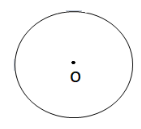Ans: The line segment joining the centre of the circle to a point on the given circle forms the radius.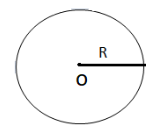The radius of the circle is R

c) A Diameter

Ans: A diameter of a circle is a chord passing through the centre of the circle.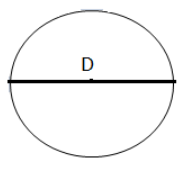D is the diameter

d) A sector

Ans: A sector is bounded by an arc on one side and a pair of radii on the other two sides.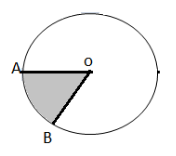OAB is a sector of the circle.

e) A segment

Ans: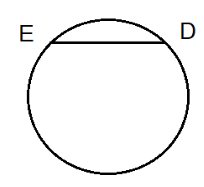In the shown diagram ED is a segment, touching the cirumferrance of the circle.

f) A point in its interior

Ans:

(Image will be updated soon)

As, shown point “C” is in interior of the circle.

g) A point in its Exterior

Ans:

(Image will be updated soon)

From the diagaram point “F” is in the exterior of the circle.

h) An Arc

Ans:

(Image will be updated soon)

In this the given Arc is ED

4. Say true or false:

a. Two diameters of a circle will certainly intersect.

Ans: True

Diameter is the largest chord passing through the centre of the circle. Since diameter always passes through the centre of the circle, two diameters will certainly intersect.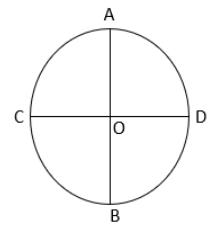Here, AB and CD are two diameters and they are intersecting at O.

b. The centre of a circle is all the time in its interior.

Ans: True

For a circle, the centre is a fixed point and the path followed from this fixed point at the same distance forms a circle.

So the centre is located always on the interior of the circle.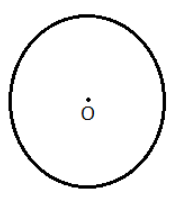The centre of the circle is O.

NCERT Maths book Class 6 Chapter 4 solutions are available on the official website of Vedantu are very easy to understand for the students. After reading the concept given in the textbook, practising solved examples, still, the students have any doubts the teachers are available on the official website to clarify those through live chat, or the students can post their doubts in the chat box. It is the ultimate advantage of NCERT solutions. Downloading PDFs is free of cost so that the students can practice some in their convenient and leisure times.

## Basic Geometrical Concepts Class 6

NCERT Solutions For Class 6 Maths Chapter 4 Basic Geometrical Ideas are given here on this page in a downloadable PDF format. It is important for the students who are looking for the top score in their final exam to practise all the questions covered in NCERT Class 6 Maths Chapter 4. While solving the questions, students might face several difficulties but the solution given in the pdf will clear all your concepts and help you to solve even the complex questions in an easy way. Therefore, students are provided here with NCERT Solutions for Class 6 Chapter 4. All the solutions to the questions given in the pdf are prepared as per the latest syllabus issued by the CBSE board.

## Topics Covered In Class 6 Maths Chapter 4

4.1: Introduction

4.2: Points

4.3: Line Segment

4.4: A Line

4.5: Intersecting Lines

4.6: Parallel Lines

4.7: Rays

4.8: Curves

4.9: Polygons

4.10:Angles

4.11: Triangles

4.13: Circles

### 4.1 Introduction

The NCERT Solutions of Class 6 Maths explains the history of geometry as it is derived from the Greek word geometry. Here geo means the earth and the matron refers to the measuring tools or measurement. The geometry is very popular and has a great history. The archaeology departments and researchers widely use it.

### 4.2 Points

This section of geometry Chapter 4 by NCERT Solutions Vedantu explains the exact definition of a point. Students can learn the difference between a dot and point as the point is the thinnest part of your Dot, and it determines the location and helps to point out some angle or any other.

### 4.3 Line Segment

The NCERT solutions of Maths Chapter 4 specify each concept of geometry with a perfect example and activity. The next topic which students have to learn is about the line segment. Students can understand that a straight line that joins two points is nothing but a line segment.

### 4.4 Aline

Many students get confused between the definition of line and line segment if the basic knowledge is not strong. That's the reason the NCERT Solutions of Vedantu provides a clear explanation to the line that has one endpoint on one side and doesn't have on the other side. In contrast, the line segment has two endpoints.

### 4.5 Intersecting Lines

The NCERT solutions of Class 6 Maths Chapter 4 have given a simple definition for the intersection of lines. According to court Solutions of Vedantu, if two lines touch at a point, then the lines are said to be intersecting lines. And the common point with which two lines are intersecting is known as the point of intersection.

### 4.6 Parallel Lines

Another important concept in the Basic Geometrical Ideas of Chapter 4 in Maths for Class 6 of NCERT Solutions in the official website Vedantu is about the parallel lines. If two lines may not have a common point and they never intersect each other, then those lines are said to be parallel lines. Students should not get confused with the concepts of intersecting lines and parallel lines.

### 4.7 Ray

The next concept of geometry chapter by the NCERT solutions of Vedantu is an array. It is not a line or line segment. Students should pay more attention to the concepts of geometry because they seem to be similar but completely different from one another. A ray is also a line, but it doesn't have any endpoint.

### 4.8 Curves

in the sections, the students can learn about the curve, which is not a straight line, and it doesn't intersect itself. It is easy to draw as well as learn.

### 4.9 Polygons

NCERT solutions of Class 6 Chapter 4 explain that the polygons are indefinite shapes formed by a group of line segments. The shape may be of any kind, but the line segment is the important element.

### 4.10 Angles

At this part of Chapter 4, the students will learn what an angle is a vast subject in the geometrical ideas. The NCERT Solutions of Vedantu specifies the definition of angle is if two rays started at a common point which forms an angle the common point is said to be the vertex, and the corner will be the angle, and the arms of lines are said to be the sides of the angle.

### 4.11 Triangles

The students are already aware of the triangles, which have three sides and three angles.

The NCERT Solutions of Vedantu explains that the quadrilateral is similar to the triangle, but it has four sides and four angles.

### 4.13 Circles

All the students can understand the concept of the circle as a closed surface which has some special properties, and it is not a polygon.

### Key Takeaways of NCERT Solutions Class 6 Maths Chapter 4 Basic Geometrical Ideas

The key takeaways of NCERT solutions of Vedantu’s official website are as follows,

• Provides the previous question papers to practise.

• The well-experienced mathematicians prepare these after thorough verification.

### Chapter wise NCERT Solutions for Class 6 Maths

Along with this, students can also view additional study materials provided by Vedantu, for Class 6 Maths.

## Importance of Class 6 Maths Chapter 4 Basic Geometrical Ideas NCERT Solutions

Geometry is an essential part of the Mathematical concepts a student learns over the years. In Class 6, students will be introduced to various new ideas of geometric elements and figures. The students will get to know new concepts and will learn to apply them to solve problems given in the exercises.

These concepts need proper elaboration along with their use in solving various crucial problems included in the chapter exercises. This is where the NCERT solutions designed by the experts will enable students to find out how to solve such problems by using the fundamental principles of geometry taught in this chapter.

With the help of these solutions, students can find out accurate answers to all the questions. They will get an idea of how to attempt to solve these questions in a stepwise method. Following these methods while practising solving such questions will help them develop their knowledge of these geometrical ideas and to utilise them for solving problems.

## Benefits of Class 6 Maths Chapter 4 Basic Geometrical Ideas NCERT Solutions

• All the exercises of this chapter have been completely solved by the experts for the convenience of studying. You can access the answers to all the questions online or can download the specific files to your computer for convenience.

• Resolve doubts related to the exercise problems and find out how to solve these questions in no time. Clarify all your doubts to complete preparing this chapter faster.

• Follow how the experts have skilfully compiled the answers to specific questions. This method will help you develop your skills and your conceptual knowledge respectively for this subject. You will become capable of answering all questions precisely and score more on the exams.

## Download Class 6 Maths Chapter 4 Basic Geometrical Ideas NCERT Solutions PDF

Get the free PDF version of these solutions and complete your study material for this chapter. Find the easiest and most accurate methods of solving these questions on your own and take your preparation to the next level. Learn to solve questions of Class 6 Maths Chapter 4 Basic Geometrical Ideas on your own and score more in the exams.

## Conclusion

NCERT Solutions for Class 6 Maths Chapter 4, "Basic Geometrical Ideas," are indispensable tools for nurturing a solid understanding of geometry among young learners. These solutions simplify complex geometric concepts and introduce students to the fundamental principles of shapes, angles, and measurements. They foster a strong foundation for advanced geometrical topics in higher grades. Furthermore, these solutions encourage critical thinking by promoting problem-solving skills, spatial reasoning, and logical deduction. Beyond the classroom, geometry plays a vital role in daily life, making these solutions not only essential for academic success but also for practical, real-world applications. Ultimately, NCERT Solutions for this chapter facilitate a holistic learning experience, equipping students with valuable geometric insights for a lifetime.

## FAQs on NCERT Solutions for Class 6 Maths Chapter 4 - Basic Geometrical Ideas

1. Describe the circle with its parts?

A circle is different from the polygon, and it is a closed surface in which the ending point comes and meets the starting point. A circle has several parts in it. If a line cuts the circle into two halves in the middle, then the line is called the diameter. Half of the diameter is nothing but the radius of the circle. Apart from the diameter, all other lines which text the circle into various parts are called chords.

2. Calculate the diameter of the circle if its radius is 4 cm?

It is given that the radius of the circle = 4 cm.

Then the diameter of the circle  = 2 * radius.

= 2 * 4

= 8 cms.

Hence the diameter of the circle is 8 cm.

3. Can I download the ebook of NCERT for Class 6 Basic Geometrical Ideas in PDF?

Yes, students can download the ebook of NCERT for Class 6 Basic Geometrical ideas online, which is available on Vedantu. Students can easily download the ebook and it can be saved on the computer. They can practice Geometry questions for scoring high marks in Class 6 Maths. NCERT Solutions for Class 6 Basic Geometrical can help students understand the basic concepts in geometry.

4. Do you also have solutions for Class 6 Basic Geometrical Ideas?

Yes, Solutions for Class 6 Basic Geometrical Ideas are available on Vedantu. Vedantu is a one-stop solution for all subjects and for any class. The solutions can help students to prepare for their exams. All solutions are given by experts so that students can understand the concepts properly. The solutions provided by Vedantu are free of cost. They are also available on the Vedantu Mobile app.

5. What are Basic Geometrical Ideas?

Geometry helps us to determine how the shapes and figures can fit together and efficiency can be maximised, and also to improve the visual appeal. The basic geometrical ideas are basic geometrical shapes, circles, lines, triangles, and quadrilaterals, curves, polygons and angles, vertices and edges definition and differences, the difference between vertical edges, triangular pyramid properties volume face edges, to calculate height width and square feet, congruency, rays, and more.